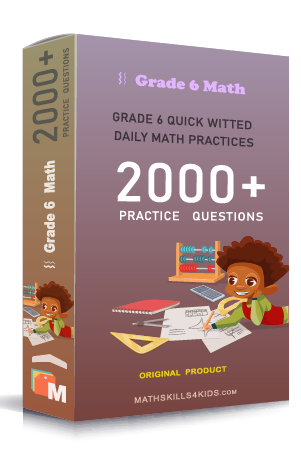# Grade 6 Percents worksheets with solutions

Subject
Math

Resource Type
Worksheets, Printables, Homeschool

Standards
CCSS 6.RP.A.3c

Format
PDF (14.3 MB | 20 pages → Exercises + Solutions)

• ### -15% OFF Over \$100

Promo Code: TOPLA

After Purchase

\$2.85

This package allows you to practice the following skills

Find the illustrated percentage

Convert between percents fractions and decimals

Compare percents to each order and to fractions

Compare percents and fractions word problems

Find percents of numbers and money amounts

Solve percents of numbers word problems

Find percents of numbers with fractional and decimal percents

Find what percent one number is of another

Find what percent one number is of another word problems

Find the total given a part and a percent

This product is a part of the Mega Pack
* Grade 6 quick-witted daily math practices *